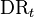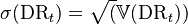# Default Rate Volatility

## Definition

Default Rate Volatility in the context of Credit Risk management denotes either

The estimation or modelling of default rate volatility inherits the characteristics of the corresponding definition of default rate:

• The length of the time interval over which default events are cumulated (mathematically can also be continuous)
• Exposure versus Count based weighting of event rates
• Cohort based volatility versus Portfolio Run rate

## Estimation Formula

The default rate volatility is simply derived from the observed variance of the default rate process$\mbox{DR}_t$$\sigma(\mbox{DR}_t) = \sqrt(\mathbb{V}(\mbox{DR}_t))$

## Volatility Models

In the context of a Credit Portfolio Model, the default rate volatility is a derived quantity, obtained from the Loss Distribution Function or related constructs